Mechanics

# Bodies in circular orbits

The astronaut has the same speed v as the space station

Let us consider the illustration below, in which we have two bodies, one of mass M and the other of mass m , such that the mass of the larger body is much greater than the mass of the smaller body, that is, M >> m . As examples of this difference in mass between bodies, we have the Sun and a planet; or a planet and a satellite. It is because of the difference between the masses of the bodies that it becomes possible for the body of mass m to rotate in a circular orbit around the body of mass M (figure below), that is, the force –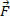has a small effect compared to the effect of strength. The poweris a centripetal force, that is, it is a force directed towards the center of the circular orbit, being then possible to calculate its intensity using Newton’s Law of Universal Gravitation.

According to Newton’s second law, we have: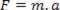Since the force we are considering is a centripetal force, we can write Newton’s 2nd Law as follows: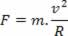Substituting the force F for the gravitational force in the above equation, and further simplifying the smaller masses, we have: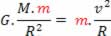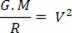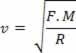Looking at the equation above, to calculate the velocity, we realize that the velocity depends on the mass of the body that is orbiting, that is, it only depends on the mass of the central body ( M ) and the radius of the orbit ( R ). Therefore, we can say that any body that rotates in the orbit of radius R will have the same speed v, since its mass is much smaller than the mass M of the central body.

This is what gives the sensation of weightlessness to an astronaut who finds himself inside a spaceship. It doesn’t matter whether the astronaut is inside or outside the spacecraft: his speed will be the same. That way, he has the sensation of floating. We call this effect weightlessness .